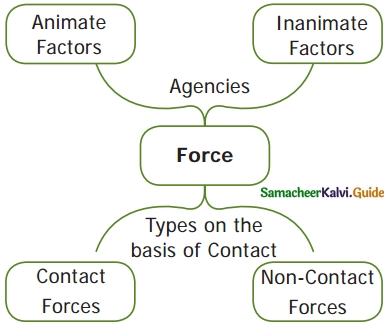Students can download 6th Science Term 1 Chapter 2 Force and Motion Questions and Answers, Notes, Samacheer Kalvi 6th Science Guide Pdf helps you to revise the complete Tamilnadu State Board New Syllabus, helps students complete homework assignments and to score high marks in board exams.

## Tamilnadu Samacheer Kalvi 6th Science Solutions Term 1 Chapter 2 Force and Motion

### Samacheer Kalvi 6th Science Force and Motion Text Book Back Questions and Answers

Question 1.
Unit of speed is
a. m
b. s
c. kg
d. m/s
d. m/s

Question 2.
Oscillatory motion among the following is
(a) Rotation of the earth about its axis
(b) Revolution of the moon about the earth
(c) To and fro movement of a vibrating string
(d) All of these
(c) To and fro movement of a vibrating stringQuestion 3.
The correct relation among the following is
a. speed = distance × time
b. Speed = distance / time,
c. speed = time / distance
d. Speed = 1 / (distance × time)
b. Speed = distance / time

Question 4.
Gita rides with her father’s bike to her uncle’s house which is 40 km away from her home. She takes 40 minutes to reach there.
Statement 1 : She travels with a speed of 1 km/minute.
Statement 2 : She travels with a speed of 1 km/hour
(a) Statement 1 alone is correct.
(b) Statement 2 alone is correct.
(c) Both Statement 1 and 2 are correct.
(d) Neither statement 1 nor statement 2 is correct.
(a) Statement 1 alone is correct

II. Find whether the following statements are true or false. – if false give the correct Answer.

1. To and fro motion is called oscillatory motion.
2. Vibratory motion and rotatory motion are periodic motions.
3. Vehicles moving with varying speeds are said to be in uniform motion.
4. Robots will replace human in future.

1. True
2. True
3. Non-uniform.
4. False, Robots can never be conscious.III. Fill in the blanks:

1. A bike moving on a straight road is an example of ……… motion.
2. Gravitational force is a ………… force.
3. Motion of a potter’s wheel is an example of ………… motion.
4. When an object covers equal distances in equal interval of time, it is said to be in ……….. motion.

1. Linear
2. non-contact
3. Rotatory
4. Uniform

IV. Match the following: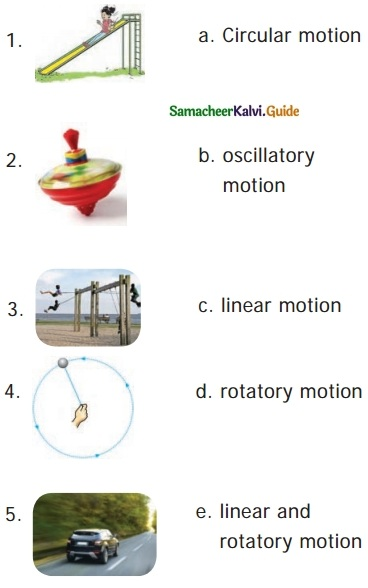1. – c
2. – d
3. – b
4. – a
5. – e

V. Analogy:

1. Kicking a ball: contact force :: falling of leaf : …………
2. Distance : metre :: Speed : ………….
3. circulatory motion : a spinning top oscillatory motion : …………

1. non contact forces
2. m/s
3. PendulumVI. Given below is the distance – travelled by an elephant across a forest with uniform speed.

Complete the data of the table given below with the idea of uniform speed.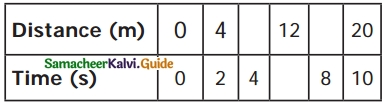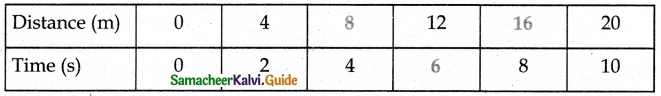VII. Complete the web chart.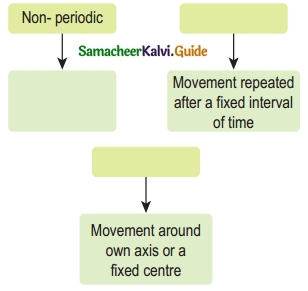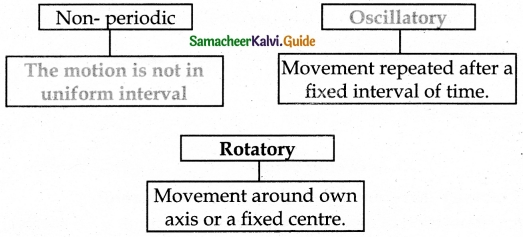VIII. Give one word for the following statements.

Question 1.
The force which acts on an object without Physical contact with it.
Non-contact force.

Question 2.
A change in the position of an object with time.
Motion.

Question 3.
The motion which repeats itself after a fixed interval of time.
Oscillatory motion.

Question 4.
The motion of an object travels equal distances in equal intervals of time.
Uniform motion.

Question 5.
A machine capable of carrying out a complex series of actions automatically.
Robots.IX. Answer the following in a sentence or two:

Question 1.
Define force.
Forces are push or pull by an animate or inanimate agency.

Question 2.
Name different types of motion based on the path.

1. Linear motion.
2. Curvilinear motion.
3. Circular motion.
4. Rotatory motion.
5. Oscillatory motion.
6. Zigzag (irregular) motion.

Question 3.
If you are sitting in a moving car will you be at rest or motion with respect to your friend sitting next to you?
I am at rest with respect to my friend, sitting inside the car.

Question 4.
The rotation of the earth is a periodic motion. Justify.
Motion repeated in equal intervals of time is called periodic motion. The earth rotates on its axis once in 24 hours. The duration of time is fixed as 24 hours. Therefore the rotation of the earth is a periodic motion.Question 5.
Differentiate between rotational and curvilinear motion

Rotational motion:

1. A body moves along a circular path.
2. Without changing its position, about its own (fixed) axis.
3. Eg. Rotation of a spinning top.

Curvilinear motion:

1. A body moves along a curved path.
2. Changes its position with motion.
3. Eg. Throwing paper airplanes or paper darts.

X. Calculate.

Question 1.
A vehicle covers a distance of 400 km in 5 hours calculate its average speed.
Average speed = $$\frac{distence}{time}$$
= $$\frac{400}{5}$$ = 80 km per hour

Question 1.
What is motion? classify different types of motion with examples.
Motion: The change of position of an object with respect to time is known as motion.

1. Based on Path:

• Linear motion. – Ex.: Parade of the soldiers.
• Curvilinear motion. – Ex.: Paper flight moving.
• Circular motion. – Ex.: Swirling stone tied to the rope.
• Rotatory motion. – Ex.: Rotating top.
• Oscillatory motion. – Ex.: Clock pendulum.
• Zigzag (irregular) motion. – Ex.: Motion of a bee.

2. Based on Duration :

• Periodic motion. – Ex.: Motion of a bob of simple Pendulum.
• Non-periodic motion. – Ex.: Swaying of the branches of a tree.

3. Based on Speed:

• Uniform motion. – Ex.: Hour hand of a clock.
• Non – uniform motion. – Ex.: Motion of a train, as it leaves a station.XII. Fill with Examples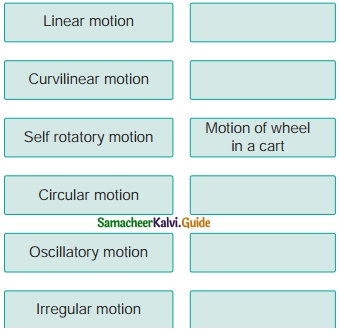Linear motion – A man walking in a straight road
Curvilinear motion – motion of paper Aeroplane
Self rotatory motion – Motion of wheel in a cart
Circular motion – Motion of moon a round earth.
Oscillatory motion – Motion of pendulum
Irregular motion – Motion of honey bee.

### Samacheer Kalvi 6th Science Force and Motion Additional Important Questions and Answers

I. Choose the best option:

Question 1.
How earth revolves?
(a) From west to east
(b) east to west
(c) From north to south
(d) south to west.
(a) From west to east

Question 2.
People walking in a crowded street is an example of _______ motion.
(a) linear
(b) circular
(c) rotatory
(d) zigzag
(d) zigzagQuestion 3.
What is the unit of average speed in SI system?
(a) metre / second
(b) kilometre/ seconds
(c) kilometre/ time
(d) Metre/ Time.
(a) metre / second

Question 4.
Usain Bolt crossed 100 metre in _______ seconds and made a world record.
(a) 9.58
(b) 9.83
(c) 9.85
(d) 9.38
(a) 9.58

Question 5.
The motion of the moon which revolves around the earth is
(a) Oscillatory motion
(b) Periodic motion
(c) curvilinear
(d) b and c
(b) Periodic motion

1. Change of position of an object with respect to time is motion
2. Swirling stone tied to the rope is an example of rotatory motion.
3. The children flying in a swing is a periodic motion.
4. All the oscillatory movements are periodic motions.
5. The SI unit of speed is kilometer

1. correct
2. wrong.
A swirling stone tied to the rope is an example of circular motion.
3. correct
4. correct
5. wrong, The SI unit of speed is meter.III. Fill up the blanks:

1. People walking in a crowded street is ……….. type of motion.
2. Object moves not at a constant speed then the motion is ………..
3. If Priya in her cycle travels 40 km in 2 hours then what is her average speed?
4. Motion is of ………… types.
5. The movement of a body about its own axis is ………….

1. Irregular
2. on- uniform motion
3. 20 km /hr
4. four
5. Rotatory motion

IV. Matching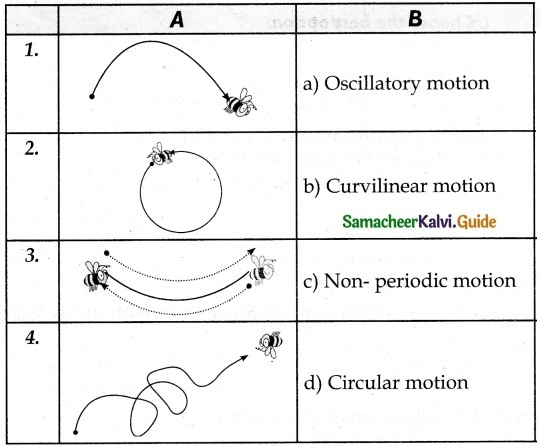1. – b
2. – d
3. – a
4. – c

V. Fill up the blanks in accordance with their relation:

1. The Motion of train – uniform motion. The motion of vehicles ………..
2. Periodic motion – The motion of the moon revolves around the earth. Oscillatory motion ………..

1. non – uniform motion.
2. Motion of simple pendulum.VI. Fill up the missing statement.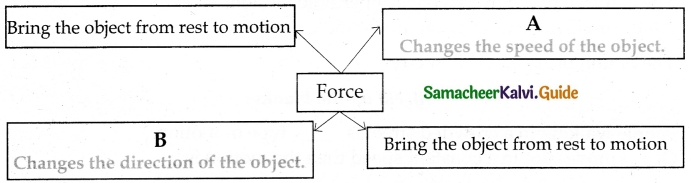VII. Fill up the blanks with the help of examples:

1. The motion of the needle of a clock.
2. The motion of an athlete of 100 m race
3. The motion of flag wagging
4. The motion of a car that goes in a circular path.
5. The motion of a needle in a sewing machine.

1. Oscillatory motion.
2. Linear motion.
3. Non- periodic motion
4. circular motion
5. periodic motion or uniform motion.

VIII. Two Mark Questions: Short answers:

Question 1.
Define motion.
When there is a change of position of an object with respect to time, then it is called motion.

Question 2.
What are the types of Force?

1. Contact Force;
2. Non-Contact Force

Question 3.
List out the types of forces on the basis of contact.
There are two types:

1. Contact force,
2. Non-contact force.Question 4.
What is the average speed of the object?
The distance travelled by an object in unit time is called the average speed of the object.

Question 5.
Define non-periodic motion.
When a motion is not in uniform interval, then such motion is called non-periodic motion.

Question 1.
What is a force? What are its types?
Forces: Forces are push or pull by an animate or inanimate agency.
Types of Forces:

1. Contact forces;
2. Non – Contact forces.

1. Contact forces: The force that is executed by touching the body is a contact force Eg. Kicking the football
2. Non – Contact forces: The force is applied. Without touching the object then it is called noncontact force.
Forces can
(i) Change the states of the body from rest to motion or motion to rest.
(ii) Either change the speed or direction or both of the body.
(iii) Change the shape of the body.